Function Repository Resource:

# NextIntegerComposition

Get the next composition of an integer in canonical order

Contributed by: Wolfram Staff (original content by Sriram V. Pemmaraju and Steven S. Skiena)
 ResourceFunction["NextIntegerComposition"][l] gives the integer composition that follows l in canonical order.

## Examples

### Basic Examples (1)

The composition of an integer following the given one:

 In:=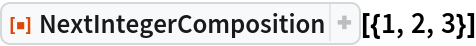Out=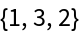### Properties and Relations (2)

NextIntegerComposition[l] gives the successor to l in the list returned by the resource function IntegerCompositions:

 In:=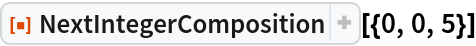Out=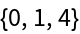In:=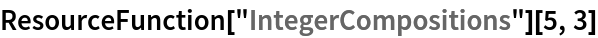Out=You can obtain this list by iteratively computing the next integer up to the length of such compositions, which for the integer n split into k parts is Binomial[n+k-1,n]:

 In:=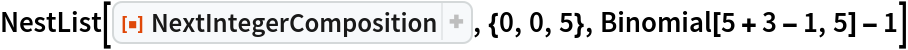Out=## Version History

• 1.0.0 – 04 March 2020